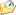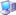* 帖子主题：ASP 全局自动关闭 数据库连接

😂 这是流量小的网站，一般看不出问题来。不过从中折射出一个问题：即使过了20来年，还是有人不喜欢做一些看起来可做可不做的事。只要不做没有出现什么问题，为什么要去做呢？

Set Db = New DbHelper
Set Conn = Db.GetConn2023/1/16 14:32:49IP：已设置保密

Set Db = new DbHelper
MsgBox "run 1: " & Db.Query("select getdate()")(0)
MsgBox "run 2: " & Db.Query("select getdate()")(0)

Class DbHelper
Private Conn, Rs

Public Function Query(sql)
If GetRs.State = 1 Then Rs.Close
Rs.Open sql, GetConn, 1, 1
Set Query = Rs
End Function

Public Function GetConn
If IsEmpty(Conn) Then
Conn.Open "Provider=Microsoft.Windows.SqlLite.OleDb.4.0; Data Source=Test.sdf"
End If
Set GetConn = Conn
End Function

Public Function GetRs
If IsEmpty(Rs) Then Set Rs = CreateObject("AdoR.RecordSet")
Set GetRs = Rs
End Function

Private Sub Class_Terminate
If Not IsEmpty(Rs) Then
If Rs.State = 1 Then Rs.Close
Set Rs = Nothing
End If

If Not IsEmpty(Conn) Then
If Conn.State = 1 Then Conn.Close
Set Conn = Nothing
End If
End Sub
End Class2023/1/16 14:41:50IP：已设置保密
pojin (ID: 2)2023/1/17 9:22:02IP：已设置保密
serverMM3 (ID: 42)2023/8/19 13:24:04IP：已设置保密